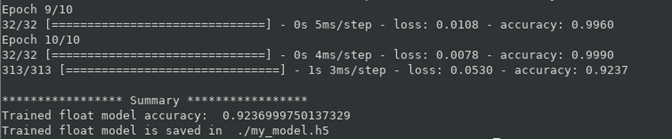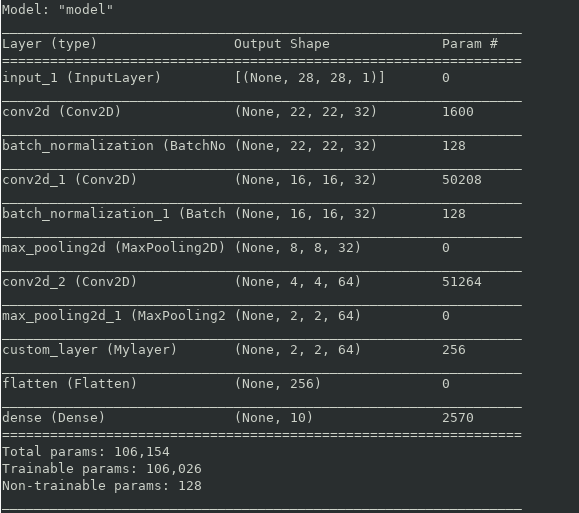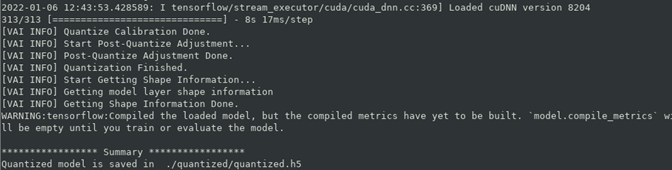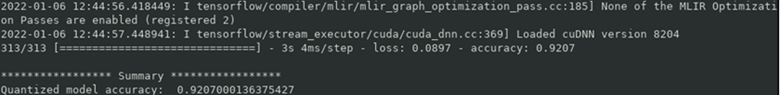# Model Quantizing - 2.0 English

## Vitis AI User Guide (UG1414)

Document ID
UG1414
Release Date
2022-01-20
Version
2.0 English

Tensorflow 2 provides a lot of common built-in layers to build the machine learning models, as well as easy ways for you to write your own application-specific layers either from scratch or as the composition of existing layers. Layer is one of the central abstractions in `tf.keras`, subclassing `Layer` is the recommended way to create custom layers. Please refer to tensorflow user guide for more information.

Vai_q_tensorflow2 provides support for new custom layers via subclassing. This tutorial will demonstrate how to quantize models with custom operations step-by-step.

Note: Custom model via subclassing `tf.keras.Model` is not supported by vai_q_tensorflow2 in this release, please flatten it to layers.

## 1. Train custom layer model

In this example, we define the custom layer named `MyLayer` to perform a "PReLU" function in train_eval.py. This custom layer performs the function below with a trainable weight `alpha`.
``````f(x) = alpha * x , if x < 0
f(x) = x , if x >= 0``````
where `alpha` is a learned array with the same shape as `x`.
Then we build a CNN model to classify the MNIST dataset as an example. Run 1_run_train.sh to train the model and you will get the float model my_model.h5 and the accuracy of the model should be >90%.
``bash 1_run_train_float.sh``This float model contains both the model structure and the weights, with a custom layer named `custom_layer`. We can get this information from the printed summary.## 2. (Optional) Evaluate the float model

You can run the script 2_run_eval_float.sh to test the trained float model.
``bash 2_run_eval_float.sh``

## 3. Quantize the float model

You can quantize the float model with custom layers using the vai_q_tensorflow2 `quantize_model` API. Example code is shown below:
``````from tensorflow_model_optimization.quantization.keras import vitis_quantize
The `custom_objects` argument must be passed into the class `VitisQuantizer` when quantizing models with custom layers. The `custom_objects` argument is a dict containing the `{"custom_layer_class_name":"custom_layer_class"}`, multiple custom layers should be separated by a comma. Moreover, `add_shape_info` should also be set to True for models with custom layers to add shape inference information for them.
During quantization, these custom layers will be kept untouched in the quantized model. Run 3_run_quantize.sh to do quantization:
``bash 3_run_quantize.sh``
If everything runs correctly, the quantized model named quantized.h5 will be generated in ./quantized/ directory. This model can be used as the input of the xcompiler and then deployed on boards.## 4. (Optional) Evaluate the quantized model

We can use `model.evaluate` API to evaluate the quantized model. Remember to recompile the model with correct losses and metrics because these information are ignored during the quantization process.
``````quantized_model.compile(loss="binary_crossentropy", metrics=["accuracy"])
quantized_model.evaluate(x_test, y_test)``````
Run 4_run_eval_quant to evaluate the quantized model.
``bash 4_run_eval_quant.sh``

It can be seen that the quantized model has close accuracy to the float model.## 5. Dump the golden results

Golden results are used to check the data correctness or debug the deployed models. Vai_q_tensorflow2 provides `dump_model` API to dump the weights/biases and intermediate activations of the quantized model with a sample input. Since the DPU dumping results are batch by batch, we need to set the batch_size of dataset to 1 when dumping golden results.
``vitis_quantize.VitisQuantizer.dump_model( model=quant_model, dataset=x_test[0:1], output_dir="./dump_results", dump_float=True)``
Since the custom layer is not quantized, we need to set `dump_float=True` to dump float weights and activation for them. Run 5_run_dump.sh to dump the quantized model.
``bash 5_run_dump.sh``
You can see the generated golden results in the folder ./dump_results. ./dump_results/dump_results_weights are the save weights, while ./dump_results/dump_results_0 are the saved activation, where the number 0 represents the index of the dataset.